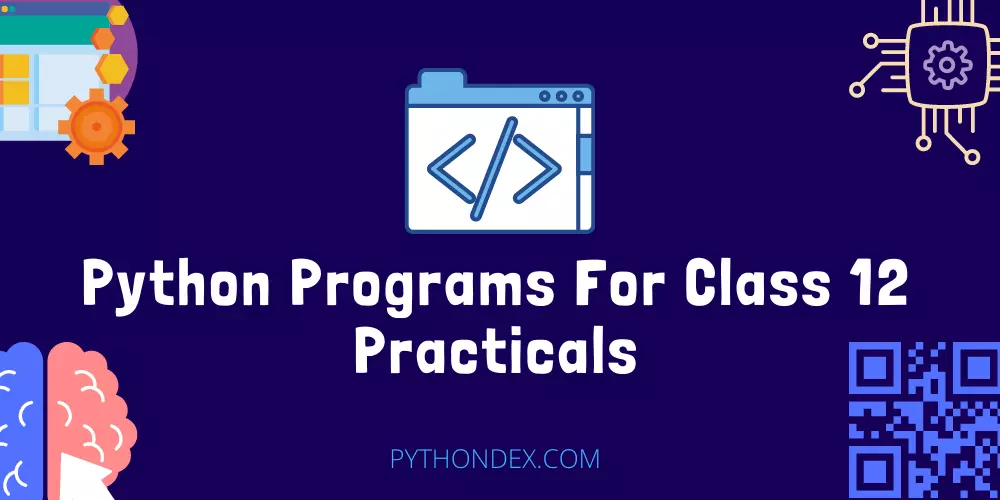ExplorePython Programs# Python Programs For Class 12 PracticalsWant some python practical programs for class 12 then today in this article I will share with you all the python programs for class 12 Practical with source code.

All the python programs in this article are for class 12 cbse students this python programs can also be used by class 11 students also.

If you want python projects for class 12 then you can refer to this article – Python projects for class 12 with source code.

## Python Programs For Class 12 Practical

All these python programs which I am going to tell you can appear for your class 12 practicals so go through each of the python programs thoroughly don’t miss any program read this article till the end.

As you read this article you can test this python program in an online compiler and see the output of each program.

Here is the list of all the class 12 python programs:

Above were all the python programs for class 12 read below for more information there will be output and explanation of each program.

### 1. Python program to print hello world

``````
print('Hello World');
```
```

Output

``````
Hello World
```
```

This is a simple python program that will print hello world in the console. The print function prints the text inside it on the console for us that was Hello World.

### 2. Python program to find maximum of two numbers

``````
def maximum(a,b):
if a >= b:
return a
else:
return b

a = 4
b = 5

print(maximum(a,b))
```
```

Output

``````
5
```
```

This python program finds the maximum of two numbers and prints the maximum number on the screen.

### 3. Python program to find factorial of a number

``````
def factorial(n):
if n < 0:
return 0
elif n == 0 or n == 1:
return 1
else:
fact = 1
while(n > 1):
fact *= n
n -= 1
return fact

num = 5;
print("Factorial of",num,"is",factorial(num))
```
```

Output

``````
Factorial of 5 is 120
```
```

This python programs finds the factorial of a given number and print the result in the console.

### 4. Python program to check even or odd numbers

``````
num = int(input('Enter a number: '))

if (num % 2) == 0:
print(f'number {num} is Even')
else:
print(f'number {num} is Odd')
```
```

Output

``````
>> Enter a number: 12
Number 12 is Even
```
```

This python program asks for a number input then the program checks if it is even or odd then prints the result.

### 5. Python program to find area of a triangle

``````
a = 5
b = 5
c = 7

s = (a + b + c) / 2

area = (s*(s-a)*(s-b)*(s-c)) ** 0.5
print('The area of the triangle is %0.2f' %area)
```
```

Output

``````
The area of the triangle is 12.50
```
```

This python program finds the area of a triangle and prints it on the console. It has the length, breadth and height given then it uses the formula to find area.

### 6. Python program to find area of circle

``````
def findArea(r):
PI = 3.142
return PI * (r*r);

print('Area of circle is %.6f' % findArea(5));
```
```

Output

``````
The area of circle is 78.550000
```
```

This python program finds the area of circle and prints it in the console window. It uses the PI and radius to find the area.

### 7. Python program to check leap year

``````
def checkYear(year):
if (year % 4) == 0:
if (year % 100) == 0:
if (year % 400) == 0:
return True
else:
return False
else:
return True
else:
return False

year = 2000
if(checkYear(year)):
print("Leap Year")
else:
print("Not a Leap Year")
```
```

Output

``````
Leap Year
```
```

This python program checks if a given year is a leap year or not then it prints out in the console.

### 8. Python program to print the Fibonacci sequence

``````
nterms = int(input("How many terms? "))

n1, n2 = 0, 1
count = 0

if nterms <= 0:
elif nterms == 1:
print("Fibonacci sequence upto",nterms,":")
print(n1)
else:
print("Fibonacci sequence:")
while count < nterms:
print(n1)
nth = n1 + n2
n1 = n2
n2 = nth
count += 1
```
```

Output

``````
>> How many terms: 5
0
1
1
2
3
```
```

This python program prints the fibonacci sequence. It asks the user for how many terms it should print then it prints all the fibonacci sequence,

## Summary

These were all the python programs for class 12 so do try and understand how they work you need to have some python knowledge to understand how these programs works.

I will be updating more python programs on this guide in the future so to get updated on when we update join our Telegram channel.

Thank you for reading, have a nice day 🙂